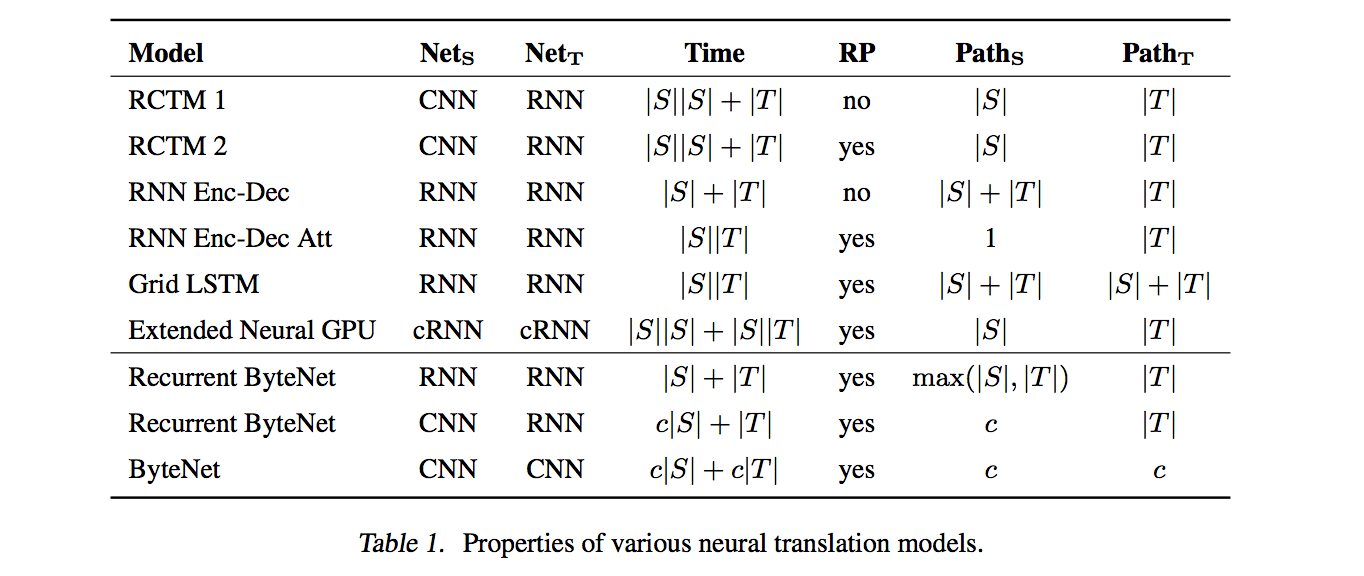# NIUHE

### Paper Reading - Neural Machine Translation In Linear Time (ByteNet)

ByteNet 可用于字符级的机器翻译模型并且有着很好的表现，它的特点在于可以在线性时间 (linear time) 完成翻译而且能够处理长距离依赖。它也采用编码器-解码器架构，并且编码器和解码器都由CNN组成。

ByteNet 之所以有上述的这些特性，是因为使用了如下一些技术：

• Dynamic Unfolding
• 解决了生成不同长度翻译的问题
• Dilated Convolution
• 缩短了依赖传播的距离
• 保证训练时只用过去的信息生成当前字符
• Residual Blocks
• 解决梯度消失问题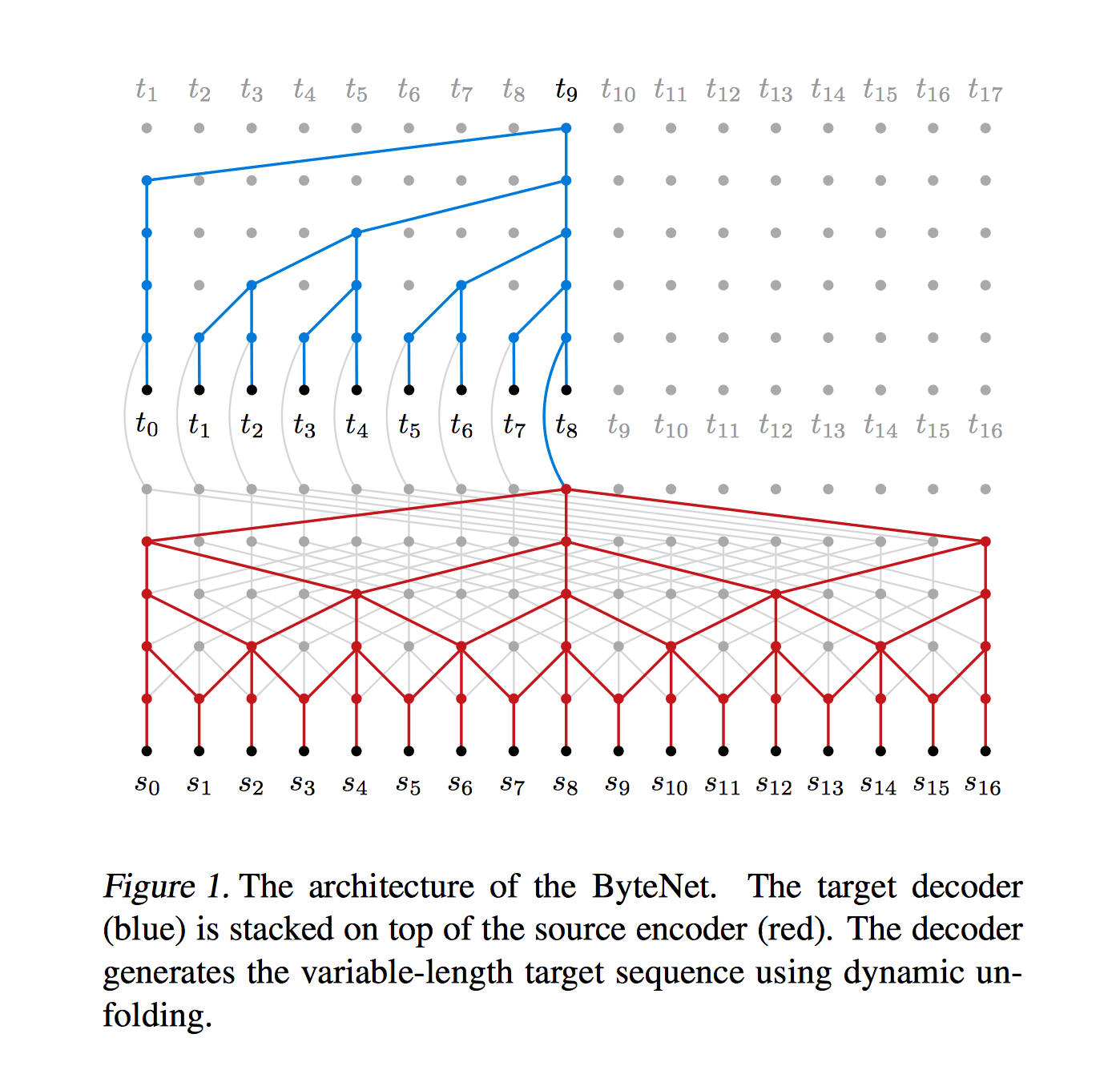## Dynamic Unfolding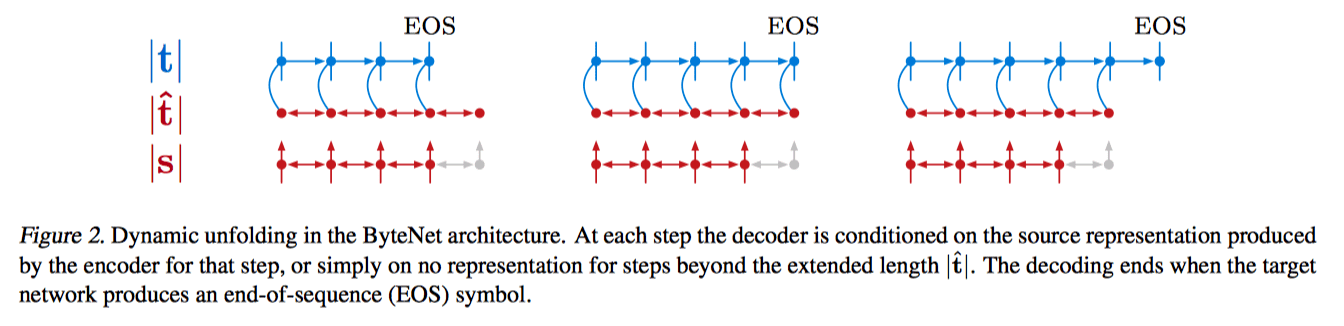Encoder 的输出的句子编码的长度固定为 $|\hat{t}|$ (不够会补零)，是目标句子长度 $|t|$ 的上界，可以通过下式得到： $|\hat{t}| = a|s| + b$ 其中，$|s|$ 表示源句子长度，通过选择适当的参数 $a$$b$，使得 $|\hat{t}|$ 基本都大于实际长度 $|t|$，并且没有太多冗余。

## Dilated Convolution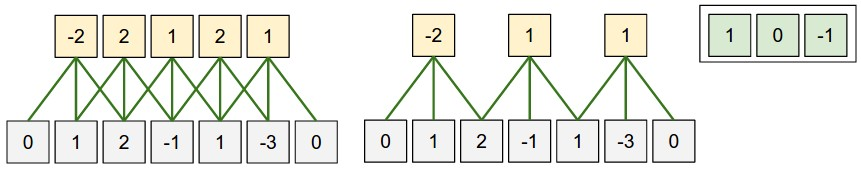Dilated Convolution的定义为： $(f*_lg)[n] = \sum_{m=-\infty}^{\infty}f[m]g[n-lm] = \sum_{m=-M}^Mf[n - lm]g[m]$ 其中，$l$ 为 dilation factor，控制扩张大小，这样 $l = 2$ 时上面例子中的卷积就变成了： $\begin{array}{lcl} (f*_2g) & = & fg + fg + fg \\ (f*_2g) & = & fg + fg + fg \\ (f*_2g) & = & fg + fg + fg \\ \end{array}$$l = 3$ 时，相应卷积就为: $(f*_3g) = fg + fg + fg$ 这样虽然卷积核都为3，但 receptive field 的大小却大了很多，所以使用 dialted conv 能使 receptive field 的大小呈指数增长，而相应参数却是线性增长的，如下图所示。使用 dilated conv 就可以有效地缩短依赖传播的距离。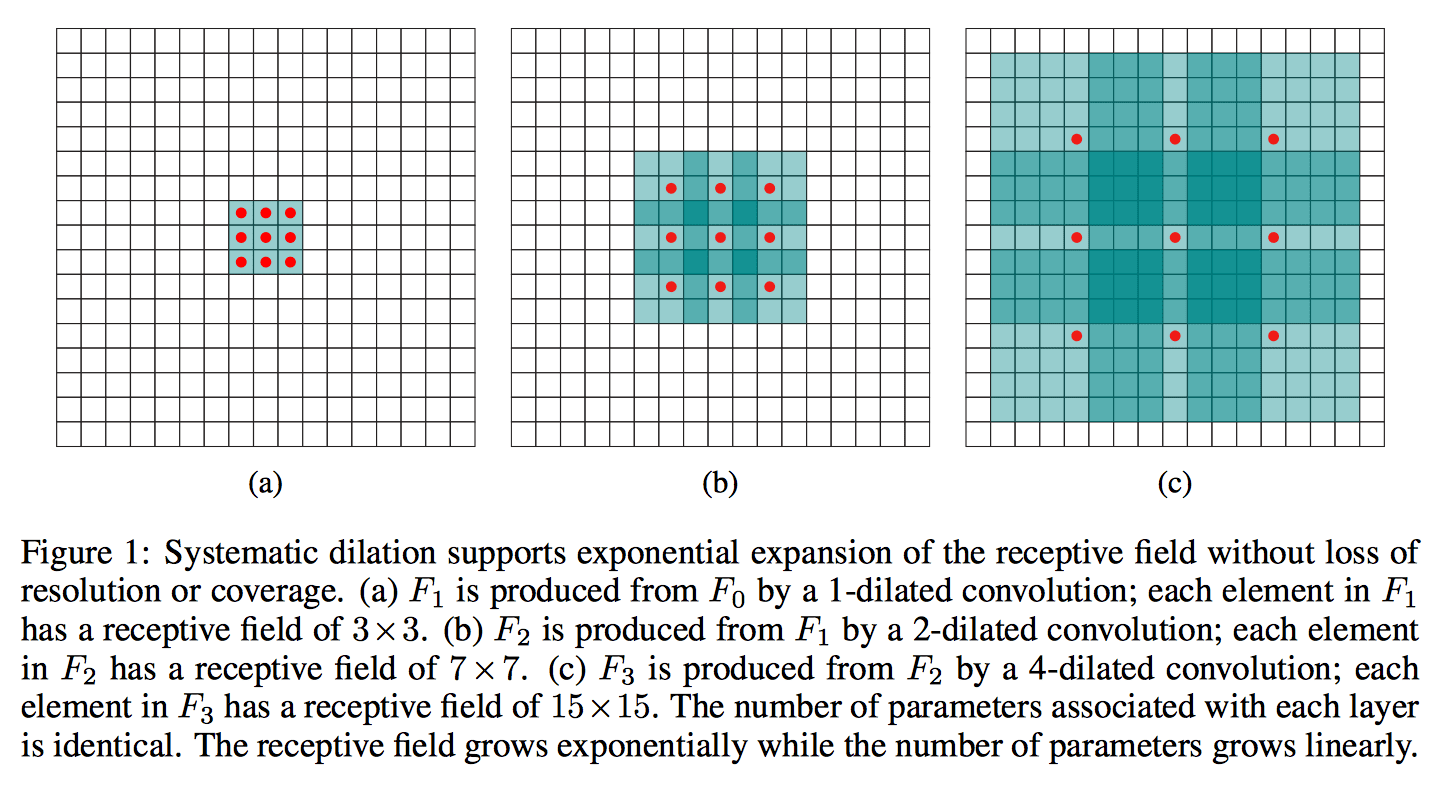## Residual Block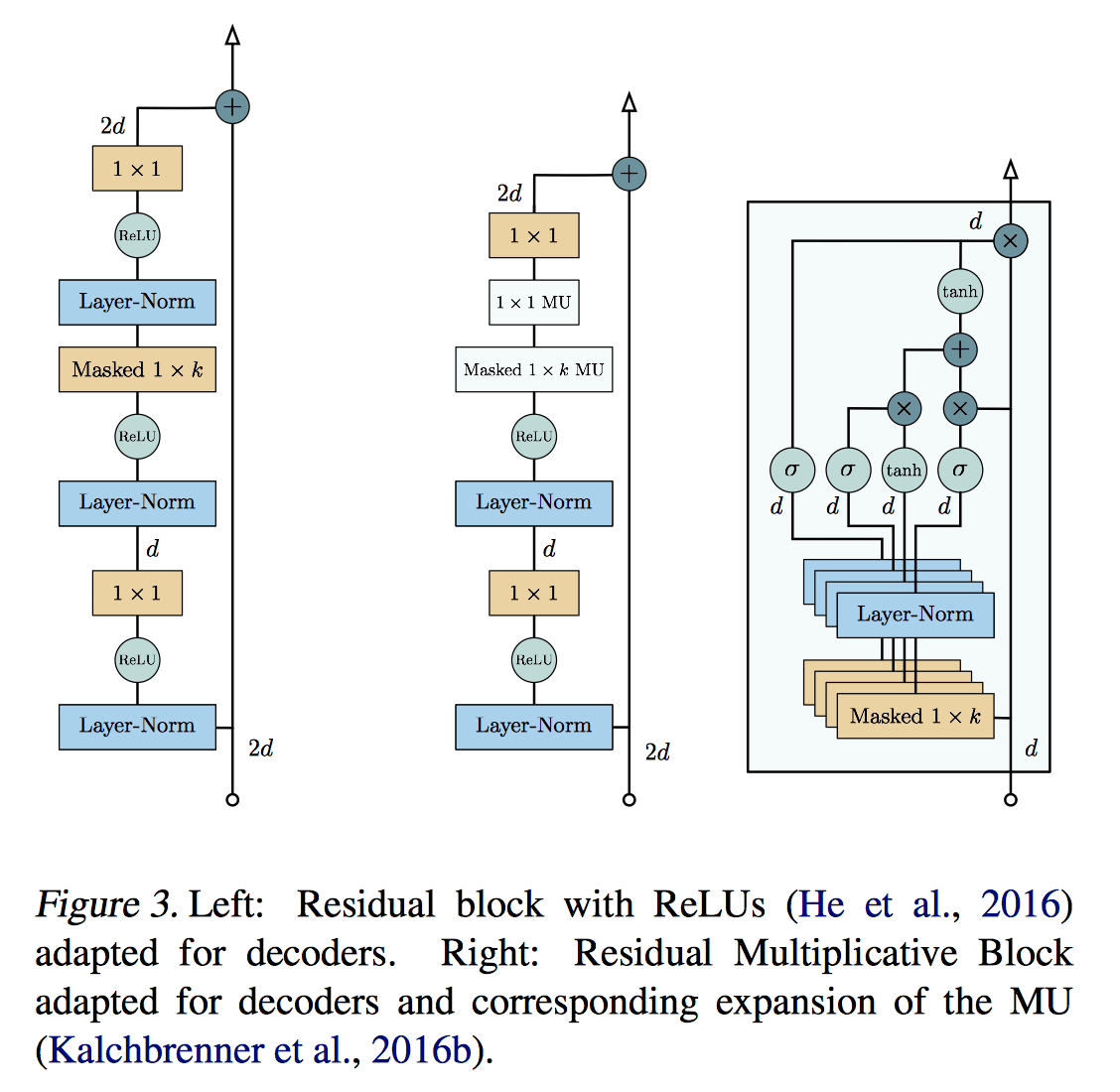## Linear Time